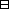## Group Theory and Linear Algebra

Last updated: 17 September 2014

## Lecture 5: Rings and Fields

An abelian group is a set $A$ with a function (addition) $A×A⟶A (a,b)⟼a+b$ such that

 (a) If ${a}_{1},{a}_{2},{a}_{3}\in A$ then $\left({a}_{1}+{a}_{2}\right)+{a}_{3}={a}_{1}+\left({a}_{2}+{a}_{3}\right)\text{.}$ (b) There exists $0\in A$ such that if $a\in A$ then $0+a=a$ and $a+0=a\text{.}$ (c) If $a\in A$ then there exists $-a\in A$ such that $a+\left(-a\right)=0$ and $\left(-a\right)+a=0\text{.}$

 (a) $ℤ$ with addition. (b) ${M}_{1×5}\left(ℝ\right)=\left\{\text{column vectors of length 5 with entries in} ℝ\right\}$ with $(a1a2a3a4a5)+ (b1b2b3b4ab)= ( a1+b1 a2+b2 a3+b3 a4+b4 a5+b5 ) .$

A ring is an abelian group $R$ with a function (multiplication) $R×R⟶R (a,b)⟼ab$ such that

 (d) If ${r}_{1},{r}_{2},{r}_{3}\in R$ then $\left({r}_{1}{r}_{2}\right){r}_{3}={r}_{1}\left({r}_{2}{r}_{3}\right),$ (e) There exists $1\in R$ such that if $r\in R$ then $r·1=r$ and $1·r=r\text{.}$ (f) If ${r}_{1},{r}_{2},{r}_{3}\in R$ then $r1(r2+r3)= r1r2+r1r3 and(r1+r2) r3=r1r3+r2 r3$ (the distributive properties).

 (a) $ℤ$ with addition and multiplication. (b) $ℤ}{mℤ}$ with addition and multiplication. (c) $ℂ\left[t\right],$ polynomials, with addition and multiplication. (d) ${M}_{n×n}\left(ℝ\right),$ square matrices, with addition and multiplication.
$ℂ[t]= { a0+a1t+a2 t2+⋯ | ai∈ℂ and all but a finite number of the ai are 0 }$ $(5t2+3t+7) (3t3+5) = 15t5+25t2+ 9t4+15t+21t3 +35 = 15t5+9t4+2 t3+25t2+15 t+35.$

A commutative ring is a ring $R$ such that

 (g) if ${r}_{1},{r}_{2}\in R$ then ${r}_{1}{r}_{2}={r}_{2}{r}_{1}\text{.}$

A field is a commutative ring $𝔽$ such that

 (h) If $r\in 𝔽$ and $r\ne 0$ then there exists ${r}^{-1}\in 𝔽$ such that $r·{r}^{-1}=1$ and ${r}^{-1}·r=1\text{.}$

 (a) $ℚ=\left\{\frac{a}{b} | a,b\in ℤ,b\ne 0\right\}$ with $\frac{a}{b}=\frac{c}{d}$ if $ad=bc\text{.}$ (b) $ℝ=\left\{\text{decimal expansions}\right\}\text{.}$ (c) $ℂ=\left\{a+bi | a,b\in ℝ\right\}$ with ${i}^{2}=-1\text{.}$

### Clocks

$1 1 2 1 32 1 42 3 1 52 43 ℤ1ℤ ℤ2ℤ ℤ3ℤ ℤ4ℤ ℤ5ℤ$ Better to write $0 0 1 0 21 0 31 2 0 41 32 ℤ1ℤ ℤ2ℤ ℤ3ℤ ℤ4ℤ ℤ5ℤ$ All of these are commutative rings. Which are fields? In $ℤ}{5ℤ}\text{:}$ $1·1=1,$ $2·3=1,$ $3·2=1,$ $4·4=1\text{.}$
In $ℤ}{4ℤ}\text{:}$ $1·1=1,$ $3·3=1,$ but $2·x$ is never $1\text{.}$
So $2$ is not invertible in $ℤ}{4ℤ},$ and $ℤ}{4ℤ}$ is not a field.

${M}_{2×2}\left(ℂ\right)$ is not a commutative ring since $(0100), (0010)∈ M2×2(ℂ)and$ $(0100) (0010)= (1000) and (0010) (0100)= (0001)$ so that $(0100) (0010) ≠ (0010) (0100).$

Let $A$ be an abelian group. Let $a\in A\text{.}$ Show that $-\left(-a\right)=a\text{.}$Proof. Assume $a\in A\text{.}$ To show: $-\left(-a\right)=a\text{.}$ We know: $-a$ is an element (call it $b\text{)}$ such that $b+a=0and a+b=0. (1)$ We know: $-\left(-a\right)=-b$ is an element (call it $c\text{)}$ such that $c+b=0and b+c=0. (2)$ To show: $c=a\text{.}$ $c=c+0=c+ (b+a)= (c+b)+a= 0+a=a,$ by properties (b), (1), (a), (2), (b), respectively. $\square$

Let $A$ be an abelian group. Show that $0\in A$ is unique.Proof. To show: $0\in A$ is unique. $0$ is an element (call it $a\text{)}$ such that $if x∈A then a+x=x and x +a=x. (3)$ Let $b$ be another element such that $if x∈A then b+ x=x and x+b=x. (4)$ To show: $a=b$ $a=a+b=b,$ by (4) and (3), respectively. $\square$

Let $R$ be a ring. Let $a\in R\text{.}$ Show that $0·a=0\text{.}$Proof. Assume $a\in R\text{.}$ To show: $0·a=0\text{.}$ $0·a=(0+0)·a= 0·a+0·a (by (b) and the distributive law).$ Add $-\left(0·a\right)$ to each side to get $0=0·a(because 0·a+(-(0·a)) =0).$ $\square$

Let $R$ be a ring. Let $a\in R\text{.}$ Show that $\left(-1\right)·a=-a\text{.}$Proof. Assume $a\in R\text{.}$ To show: $\left(-1\right)·a=-a\text{.}$ We know: $-a$ is an element (call it $b\text{)}$ such that $b+a=0and a+b=0.$ We know: $-1$ is an element (call it $c\text{)}$ such that $c+1=0and 1+c=0.$ To show: $c·a=b\text{.}$ $c·a+a = (c+1)a= 0·a=0,and a+c·a = (1+c)a= 0·a=0.$ Then $b=b+0=b+a+c·a =0+c·a=c·a.$ $\square$

## Notes and References

These are a typed copy of Lecture 5 from a series of handwritten lecture notes for the class Group Theory and Linear Algebra given on August 3, 2011.